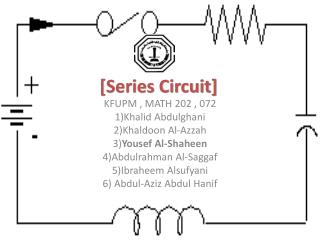DownloadDownload Presentation[Series Circuit]

[Series Circuit]

Télécharger la présentation[Series Circuit]

- - - - - - - - - - - - - - - - - - - - - - - - - - - E N D - - - - - - - - - - - - - - - - - - - - - - - - - - -
Presentation Transcript

1. [Series Circuit] KFUPM , MATH 202 , 072 1)Khalid Abdulghani 2)Khaldoon Al-Azzah 3)Yousef Al-Shaheen 4)Abdulrahman Al-Saggaf 5)IbraheemAlsufyani 6) Abdul-Aziz Abdul Hanif

2. Engineering interest !! Voltage current & Inductors Capacitors resistors

3. The problem For resistors For capacitors and inductors the current and voltage are dependent of each other and functions of time . Ohms law can be applied easily by V = ( I ) x (R)

4. capacitors the current through the capacitor depends on the change of voltage across its terminals I C = C dv / dt

5. Inductors The voltage across the inductor depends on the change of the current passing through it V = L d i / dt

6. Series circuit analysis

7. Series circuit analysis

8. The solution

9. Converting the DEs into matrices

10. We solve it by applying KCL, KVL By KVL In loop (1): ………… (1) Dividing by L1: ………… (2) Now, we apply KCL in (2) Yields, “First Eq.”

11. By KVL : In loop (2) “The outer loop” ……. (3) Dividing by L2 ………. (4) Applying KCL ,then substitute in (4) Taking i3 as common factor “second Eq.”

12. Solving the DE using MATLAB #33/P359: We have the system of DE

13. function [t,i] = ES t_interval =[0 4]; initial_condition=[0 0]; [t,i] = ode45(@rhs,t_interval,initial_condition); plot(t,i(:,1),'-',t,i(:,2),'-.') legend('i_1','i_2','Location','eastoutside'); sprintf(' t i1 i2') [t,i]

14. function di = rhs(t,i) di = zeros(2,1); % a column vector di(1) = -11*i(1) + (3/1)*i(2) + ((100*sin(t))/1); di(2) = (3/1)*i(1) - (3/1)*i(2) + 0;

15. The solution of the problem

16. The solution of the problem t i1 i2 0 0 0 0.0073 0.0026 0.0000 0.0145 0.0100 0.0001 0.0218 0.0219 0.0005 0.0290 0.0381 0.0011 0.0363 0.0580 0.0021 0.0436 0.0815 0.0036 0.0508 0.1083 0.0055 0.0581 0.1382 0.0081 0.0676 0.1814 0.0123 0.0771 0.2289 0.0177 0.0865 0.2805 0.0244

17. Thank you very much